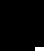Performance simulations and real life tests.

Please note, simulations described on this page were done for my vehicle before the lead acid battery pack was replaced with LiIon one.
The page will be updated when LiIon baattery model will be available.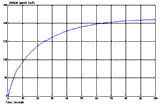Anyone, who plans to convert a vehicle to an electric one, wonders what the performance will be. Many factors influence that; some are constants we can't change, like air density or gravitational acceleration constant. These can be found in physics books. Other parameters, such as drag coefficient or gear ratios can be influenced by a vehicle designer, but usually we stuck with what the available donor vehicle is. Yet other parameters, like rolling resistance or driving technique we can change. Accurate modeling if real life performance will take giant amount of computing power and very sophisticated mathematical models of many components. Yet, end result will not be any more accurate than the worst assumption. The driving technique of the human being is not possible to model at all (it depends on the driver's mood at the moment, road conditions, whether he/she is in a hurry, etc). So usually only the hardware itself gets modeled at very simplified conditions. This proved to be accurate enough for practical purposes and proper selection and comparison of the drive train components. In my case performance was simulated based on the mathematical model of the motor, power inverter, gear box, Optima battery and also based on the other parameters taken into account (motor, inverter and gear box efficiency, battery voltage, rolling resistance and others). Currently I no longer use Optima lead acid battery pack.

The vehicle properties can be found in manufacturer's specifications.

First - about the vehicle weight. After measurements on the truck scales, total weight was 1536 kg (3,380 LB) -71% increase  compared to stock dry weight of 894 kg (1967 lb).

Summarized statistics:

 Before conversion After conversion Remark Total mass =894 kg (1967 lb) 1536 kg (3,380 lb) 71% gain Weight distribution front/rear =62%/38% =58%/42% 4% shift toward rear Front on scales = 536 kg (1,180 lb) =882 kg (1,940 lb) 65% gain Rear on scales =357 kg (786 lb) =654 kg (1,440 lb) 83% gain Left side on scales =447 kg (984 lb) =741 kg (1,630 lb) 66% gain Right side on scales =447 kg (984 lb) =795 kg (1,750 lb) 78% gain Front left wheel load (calculated) =277 kg (609 lb) =427 kg (940 lb) 54% gain Front right wheel load (calculated) =277 kg (609 lb) =455 kg (1000 lb) 64% gain, 10% more than on left side Rear left wheel load (calculated) =170 kg (374 lb) =314 kg (690 lb) 85% gain Rear right wheel load (calculated) =170 kg (374 lb) =341 kg (750 lb 100% gain, 15% more than on left side

Since 10 Optimas were laid on the passenger side floor, of course the weight distribution side to side is unequal now. But, on top of the fact that my CRX now performs better than stock one, this doesn't really bother me. New new coil springs were winded to order per this weight specifications, so they are a bit longer on the right side. Besides restoring stock ride height, the car is perfectly leveled now. With cheesy stock springs the car rides so low that I have trouble with speed bumps and drive ways entries, not to mention that it's impossible to insert a jack under car without being *very* creative :-). Low height might look cool for some, but is really shortening life of CV joints working at totally wrong angles and other suspension geometry is really skewed. I may admit that my definition of being "cool" is not the most progressive one, but it serves me well so far.

So, the Final parameters used to simulate my CRX were these:

 Vehicle mass =854 kg; Battery mass =600 kg; Driver mass =80 kg Air drag coefficient =0.29 Vehicle frontal area =1.86 m^2 Air density at 25'C =1.25 kg/m^3 Gravimetric acceleration =9.8  m/s^2 First gear reduction ratio =3.250 Second gear reduction ratio =1.650 Third gear reduction ratio =1.033 Forth gear reduction ratio =0.823 Fifth gear reduction ratio =0.694 Final differential reduction ratio =2.954 Motor shaft speed to downshift =2000 Motor shaft speed to upshift =5500 Average gear box efficiency =0.95 Rolling resistance coefficient =0.01 Wheel radius =0.28 Mass moment of inertia of all four wheels =3 kgm^2 Max motor current =280 A rms DC input =27 Batteries Optima D950S Battery OCV =13.25 V Battery SOC =1 Battery  R_int. =3 mOhm Battery pack voltage at max. motor current =334.6 V Max motor torque =195 Nm Max motor power =83.6 kW Average motor/inverter efficiency =0.86 Mass moment of inertia of transmission rotating parts =0.11 kgm^2

The functions I was interested in were:

- Acceleration rate (vehicle speed as a function of time)
- Motor shaft rotation speed as a function of time
- Battery power as a function of vehicle speed
- Driving range

Initial simulation results did not agree with actual performance and showed that in reality car should perform better than it actually does. Further research of the vehicle modes revealed that the internal resistance of the battery cables and connectors was not factored in. Actual measurements showed that while it is only a tad above 0.1 Ohm, at 50A current draw it accounts for 5V voltage drop. The other resistance is internal battery resistance totaling 0.1 Ohm for the pack as well, so the voltage sag for the whole pack (cabling included) with SOC=1 is 10V per every 50A. This alone accounts for a little more current draw than the model predicts and accordingly a bit less range.

There is almost nothing I could do with air drag which is pretty much predetermined by the body shape. I use a belly pan and that's about it. Besides air drag becomes major player at higher (highway) speeds only. A rolling resistance is main concern at any speed and something I can improve. Updated actual rolling resistance measurement done in opposite directions at low speed (30 mph) revealed that ACRX draws 14.7A average at 326VDC pack voltage. This amounts to 4,792 W of power. If I'd drive like that for 1 hour, I'd cover 30 miles and spend 4,792 Wh. So, energy consumption, then, is 4,792 Wh / 30 miles = 159.7 Wh/mile. With 14,600 Wh of useable energy on board (20% SOC) theoretically this would give me 14,600 Wh / 159.7 Wh/mile=91.4 miles range.

Not bad, but there are ways to improve it: good LRR tires, zero toe in front and rear alignment, right transmission oil to name a few.

I simulated maximum performance (2 min run) and performance in standard 10 min US06 driving cycle. For maximum acceleration I found out that there is no benefit to rev the motor above 5500 rpm because above that useable torque at the wheels falls to the lower value than that on the next gear up and accordingly lower RPM but higher torque of the motor. So I limited gear up-shifting RPM to 5500. Down-shifting is done when RPM falls below 2000. Results are presented here: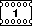Maximum performance, Velocity and motor RPM vs. time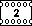Maximum performance, Total distance and Energy consumed vs. time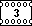Maximum performance, Battery voltage and SOC vs. time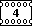Maximum performance, Battery power, current and motor shaft mechanical power vs. time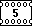Maximum performance, Battery power and Energy required vs. speed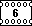US06 performance, Velocity and motor RPM vs. time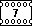US06 performance, Battery power, current and motor shaft mechanical power vs. time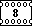US06 performance, Battery voltage and SOC vs. time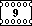US06 performance, Total distance and Energy consumed vs. timeMy 4.5 min long stop-n-go run. You can see all the benefits of regenerative braking.The same as above, the top plot is substituted for the motor RPM , instead of accel and brake usage.The plot segment of the drive on freeway. You can see the voltage rise when regen is applied (I_brake purple line on the top plot). Nice!